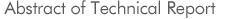No. 1201
Simulation Technique on Magnetic ApplicationsInvestigating Committee on Simulation Technique for Magnetic Applications
 Keyword : Magnetic device, Electric Motor, Switched Mode Power Supply, Finite Element Analysis, Spatial Network Analysis
In recent years, remarkable developments have been achieved in the magnetic devices such as a transformer, an inductor and electric motors. For optimum design of the magnetic devices, a precise analysis considering nonlinear magnetic characteristics of magnetic hysteresis and iron losses is important. Furthermore, a coupling analysis of magnetic-field and circuit is needed in the magnetic application systems such as a switched mode power supply and a motor drive system. From these points of view, we investigate a recent progress of the simulation technique in the magnetic devices and systems. In this technical report, we describe (1)trends of finite element analysis of various magnetic phenomena, (2)demonstration of 3-D finite element analysis of rotating machine, (3)spatial network analysis based on magnetic circuit, electric circuit and transmission circuit, (4)application of the spatial network analyses to magnetic device simulations, (5)ac-dc converter analysis using a general purpose simulation tool "Matlab/Simulink", dc-dc converter analysis using "Mathematica" and dc-ac converter analysis using "Spice", (6)a motor analysis using a spatial network analysis based on magnetic circuit, (7)an evaluation method of magnetic materials for electric motors, (8)introduction of a general purpose circuit simulator "Spice" and "Simplorer".

©2007. The Institute of Electrical Engineers of Japan